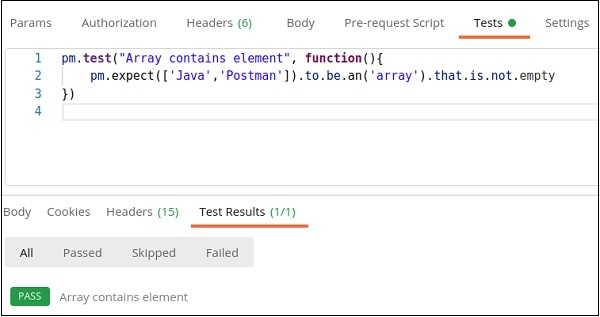# Postman - Assertion

Assertions are used to verify if the actual and expected values have matched after the execution of a test. If they are not matching, the test shall fail and we shall get the reason for failure from the output of the test.

An assertion returns a Boolean value of either true or false. In Postman, we can take the help of JavaScript Chai Assertion Library to add assertions in our tests. It is available in the Postman application automatically.

The Chai − Assertions are easily comprehensible as they are defined in a human readable format. The Assertions in Postman are written within the Tests tab under the address bar.

The documentation for Chai is available in the following link −

https://www.chaijs.com/## Writing Assertions

Let us write an assertion to check if a particular text − Postman is within an array of strings.

## Example

pm.test["Text is present"], function(){
pm.expect(['Java', 'Postman']).to.include('Postman')
})


## Output

The output is as follows −Let us write an Assertion to check if an array is empty.

## Example

pm.test["Array contains element"], function(){
pm.expect(['Java', 'Postman']).to.be.an('array').that.is.not .empty
})


## Output

The output is as follows −## Assertion for Object Verification

Let us write an Assertion for object verification with eql. It is used to compare the properties of the object i and j in the below example.

## Example

pm.test("Equality", function(){
let i = {
"subject" : "Postman"
};
let j= {
"subject" : "Cypress"
};
pm.expect(i).to.not.eql(j);


## Output

The output is as follows −The property defined for object i is Postman while the property defined for j is Cypress. Hence, not.eql Assertion got passed.

## Assertion Types

In Postman, we can apply assertions on different parts of Response. These are explained below −

### Status Code

The assertion for status code is as follows:pm.test["Status Code is 401"], function(){
pm.response.to.have.status(401)
})


The above assertion passes if the Response status code obtained is 401.

pm.test["Status is Forbidden"], function(){
pm.response.to.have.property('status', ' Forbidden')
})


The above assertion is applied on the Response property – status having the value Forbidden.

### Time taken by Response

The assertion for time taken by response is as follows −

pm.test("Response time above 500 milliseconds", function () {
pm.expect(pm.response.responseTime).to.be.above(500)
})


The above assertion passes if the Response time is above 500ms.

### Type of Response Format

The assertion for type of response format is as follows −

pm.test("Response type is JSON", function(){
pm.response.to.be.json;
})


The above assertion passes if the Response is of JSON type.

The assertion for header of response is as follows −

pm.test("Header Content-Encoding is available", function () {
})


The above assertion passes if the Response has a header Content-Encoding.

### Text of Response

The assertion for text of response is as follows −

pm.test("Response Text", function () {
pm.expect(pm.response.text()).to.include("Tutorialspoint")
})


The above assertion passes if the Response text contains the text Tutorialspoint.